Written by Chau Pham on Feb 26, 2020

The best strategy to perform vector addition is to break it into 3 components along the x,y,z axes.

To find a component of a vector is to project the vector on an axis, or resolving the vector. This can be achieved using geometry from the right triangle. ax = acos(θ), ay = asin(θ)Once a vector has been resolved into its components along a set of axes, we can take the sums of the components along each axis.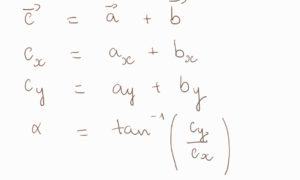You can also add vectors by placing the next vector with its tail at the previous vector’s head.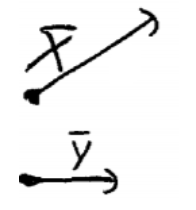We have 2 vectors x and yPlacing the tail of y at the vector x’s head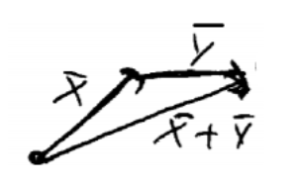Drawing a vector from the tail of the first vector to the head for the last vector. We have our resulting vector with its magnitude and direction.

You can do scalar multiplication of vectors by multiplying its magnitude and maintaining its direction.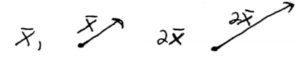Subtraction of vectors can be easily achieved by adding a vector with another vector in opposite direction.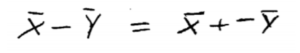KEY TAKEAWAYS

Key Points:

• Sine: think perpendicular. Cosine: think parallel
• Resolving the vector: using geometry from right triangle to find the vector’s components
• Add the vectors by drawing them with tail connected to the previous vector’s head. Drawing the resulting vector from where you start to where you end.

Key Terms:

• Tail of vector: where you start to draw a vector
• where you end

Billing Information